# Discount Questions with Solutions for Competitive Exams

Discount Questions with Solutions for Competitive Exams. Important and repeated question with answer and solution for SSC CGL, CPO, CHSL, IBPS Bank, NRA CET, UPSSSC PET and other govt jobs examinations.

### Successive Discount : Questions with Solutions

Short Trick Formula Equivalent single discount for two successive discounts of x% and y%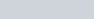$( x +y - \frac {x \times y} {100})$ %

Q.1: Successive discounts of 20% and 10% are equivalent to a single discount of :
a) 15%
b) 28%
c) 25%
d) 30%

Ans : b) 28%
Let Price is 100
New price after 20% discount = 80
10% discount on 80 = 8,
Price after both discount = 80-8 =72
Total discount = 100-72 = 28%
Alternate method
20 + 10% of 80 = 28%
Short Trick
Formula for successive discount x and y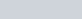$x +y - \frac {x \times y} {100}$%
=>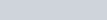$20 + 10 - \frac {20 \times 10}{100}$= 28%

Q.2: A single discount equivalent to the series of discounts 20%, 10% and 5% is equal to :
a) 32%
b) 30%
c) 30.7%
d) 31.6%

Ans : d) 31.6%
20 + 10% of 80 = 28%
28 + 5% of 72 = 28+3.6 = 31.6%
Trick$20 + 10 - \frac {20 \times 10}{100}$= 28%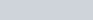$28 + 5 - \frac {28 \times 5}{100}$= 31.6%

Q. 3: A store has an offer ‘Buy 4 Get 1 Free’. What is the net percentage of discount?
a) 10%
b) 15%
c) 20%
d) 23%

Ans: c) 20%
Let price of one item is 100
Offer Price of 5 items ‘Buy 4 Get 1 Free’ = 4 + 1 = 400+0 = 400
One item price in offer = 400/5 = 80
Discount = 100 – 80 = 20%

Q.4: A scooter is sold at three successive discounts of 10%, 5% and 2%. If the marked price of the scooter is ₹18,000, find its net selling price.
a) ₹ 15,028.20
b) ₹ 15,082.00
c) ₹ 15,082.20
d) ₹ 15,080.00

Ans : c) ₹ 15,082.20
Price after 10% discount = 18000 – 1800 = 16200
Price after 5% discount = 16200 – 810 =15390
Price after 2% discount = 15390 – 307.80 =1582.20
Short Trick Formula
SP = MP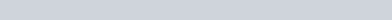$(1-\frac {\text {first discount}}{100}) \times (1-\frac {\text {second discount}}{100}) \times (1-\frac {\text {third discount}}{100})$

Q.5: A sofa-set listed at ₹800 is sold to a retailer at successive discounts of 25% and 15% by the wholesaler. Then the cost price of the sofa-set for retailer is :
a) ₹500
b) ₹510
c) ₹550
d) ₹560

Ans : b) ₹510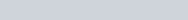$800 \times (1-\frac {25}{100})\times (1-\frac{15}{100})$
=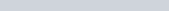$800 \times 0.75 \times 0.85 = 510$

### Finding the Loss/Gain Percentage when Market Price is x% higher of CP and Discount of y% is given on the Market Price

Q.6: A shopkeeper marks his goods 20% higher than the cost price and allows a discount of 5%. The percentage of his profit is :
a) 15%
b) 20%
c) 10%
d) 14%

Ans : d) 14%
Let CP is 100
Marked price is 120
5% discount on 120 = 6
New Price = 120 – 6 = 114
Profit percentage = 114-100 = 14%
Trick :
Gain % =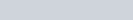$20 - 5 - \frac {20\times5}{100} = 14$%

Q.7: The marked price is 20% higher than cost price. A discount of 20% is given on the marked price. By this type of sale, there is :
a) 4% loss
b) 2% loss
c) No loss no gain
d) 4% gain

Ans : a) 4% loss
Gain percentage =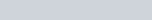$20 - 20 - \frac {20 \times 20}{100}= -4$%

### A Shopkeeper Earns a Profit of x% after allowing a Discount of y% on the Printed Price

Q.8: A man allows a discount of 10% on a book whose marked price is ₹40. What is the cost price so that the profit is 20%?
a) ₹ 35
b) ₹ 40
c) ₹ 30
d) ₹ 45

Ans : c) ₹ 30
120% of CP = 90% of 40
x= 40

Q.9 : After allowing 10% discount, a dealer wishes to sell a machine for ₹2700. At what price must the machine be marked?
a) ₹ 2700
b) ₹ 2870
c) ₹ 2460
d) ₹ 3000

Ans : d) ₹ 3000
90% of CP = 2700
CP = 3000

Q.10: A merchant offers 8% discount on all his goods and still makes a profit of 15%. If an item is marked ₹ 250, then its cost price is :
a) ₹180
b) ₹200
c) ₹230
d) ₹187

Ans : b) ₹200
92% of MP = 115% of CP
92 x 250 = 115 x CP
CP = ₹200

### Discount Mixed Problems on Marked Price : Questions with Solutions

Q.11: The marked price of a CD is ₹ 250. It is sold for ₹225. The rate of discount is:
a) 2.5%
b) 10%
c) 25%
d) 11.12%

Ans : b) 10%
Discount = 250 – 225 = 25
25 discount on 250 = 10%

Q.12: A seller gains 20% profit even after allowing 10% discount, if the amount of profit on a TV set is ₹750, then the marked price of the TV set is :
a) ₹ 5200
b) ₹ 5000
c) ₹ 4800
d) ₹ 5500

Ans : b) ₹ 5000
20% of CP = 750, CP = 3750
90% of Marked Price = 120% of 3750 (CP)
Marked Price =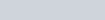$\frac {3750 \times 120}{90} = 5000$

Q.13: The marked price of an item is twice the cost price. For a gain of 15%, the discount should be:
a) 7.5%
b) 20.5%
c) 32.5%
d) 42.5%

Ans : d) 42.5%
CP is 100 , MP = 200
Selling price for gain of 15% = 115
Discount = 200 – 115 = 85
85 of 200 = 42.5%

Q.14: If a discount of 20% on the marked price of a shirt saves a man ₹150, how much did he pay for the shirt?
a) ₹ 600
b) ₹ 650
c) ₹ 500
d) ₹ 620

Ans : a) ₹ 600
Marked price = 100
Price after 20 % discount = 80
Save 20 on 100. Save 1 on 5
save 150 on marked price ₹750
Pay = 750 -150 =₹600

Q.15: A trader marked the price of his commodity so as to include a profit of 25%. He allowed a discount of 16% on the marked price. His actual profit was
a) 5%
b) 9%
c) 16%
d) 25%

Ans : a) 5%
Cost Price = 100
Marked Price = 125
Selling price 84% of 125 = 105
Profit = 105-100 = 5%

Q.16: A fan is listed at ₹1400 and the discount offered is 10%. What additional discount must be given to bring the net selling price to ₹1200?
a)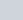$16\frac23$%
b) 5%
c)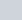$4\frac{16}{21}$%
d) 6%

Ans: c)$4\frac{16}{21}$%
Second discount % =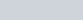$\frac {60\times100}{1260} = 4\frac {16}{21}$%